1. /
2. CBSE
3. /
4. Class 07
5. /
6. Mathematics
7. /
8. NCERT Solutions for Class...

# NCERT Solutions for Class 7 Maths Exercise 6.4### myCBSEguide App

Download the app to get CBSE Sample Papers 2023-24, NCERT Solutions (Revised), Most Important Questions, Previous Year Question Bank, Mock Tests, and Detailed Notes.

NCERT solutions for Maths Triangles and its Properties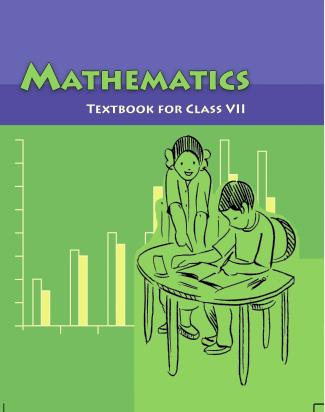## NCERT Solutions for Class 7 Maths Triangles and its Properties

###### Question 1.Is it possible to have a triangle with the following sides?
1. 2 cm, 3 cm, 5 cm
2. 3 cm, 6 cm, 7 cm
3. 6 cm, 3 cm, 2 cm

Since, a triangle is possible whose sum of the lengths of any two sides would be greater than the length of third side.

(i) 2 cm, 3 cm, 5 cm

2 + 3 > 5 No

2 + 5 > 3 Yes

3 + 5 > 2 Yes

This triangle is not possible.

(ii) 3 cm, 6 cm, 7 cm

3 + 6 > 7 Yes

6 + 7 > 3 Yes

3 + 7 > 6 Yes

This triangle is possible.

(iii) 6 cm, 3 cm, 2 cm

6 + 3 > 2 Yes

6 + 2 > 3 Yes

2 + 3 > 6 No

This triangle is not possible.

NCERT Solutions for Class 7 Maths Exercise 6.4

###### Question 2.Take any point O in the interior of a triangle PQR. Is: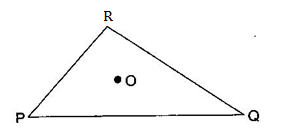1. OP + OQ > PQ ?
2. OQ + OR > QR ?
3. OR + OP > RP ?

Since sum of two sides is greater than third side.

Join OR, OQ and OP.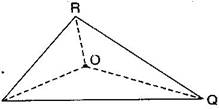(i) Is OP + OQ > PQ ?

Yes, POQ form a triangle.

(ii) Is OQ + OR > QR ?

Yes, RQO form a triangle.

(iii) Is OR + OP > RP ?

Yes, ROP form a triangle.

NCERT Solutions for Class 7 Maths Exercise 6.4

###### Question 3.AM is a median of a triangle ABC. Is AB + BC + CA > 2AM ?

(Consider the sides of triangles ABM and AMC.)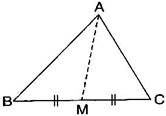Since, the sum of lengths of any two sides in a triangle should be greater than the length of third side.

Therefore, In {tex}\Delta {/tex}ABM, AB + BM > AM ……….(i)

In {tex}\Delta {/tex}AMC, AC + MC > AM ……….(ii)

AB + BM + AC + MC > AM + AM

{tex} \Rightarrow {/tex} AB + AC + (BM + MC) > 2AM

{tex} \Rightarrow {/tex} AB + AC + BC > 2AM

Hence, it is true.

NCERT Solutions for Class 7 Maths Exercise 6.4

###### Question 4.ABCD is a quadrilateral. Is AB + BC + CD + DA > AC + BD ?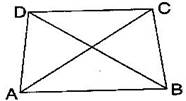Since, the sum of lengths of any two sides in a triangle should be greater than the length of third side.

Therefore, In {tex}\Delta {/tex}ABC, AB + BC > AC ……….(i)

In {tex}\Delta {/tex}DCB, DC + CB > DB ……….(iii)

Adding eq. (i), (ii), (iii) and (iv),

AB + BC + AD + DC + DC + CB + AD + AB > AC + AC + DB + DB

{tex} \Rightarrow {/tex} (AB + AB) + (BC + BC) + (AD + AD) + (DC + DC) > 2AC + 2DB

{tex} \Rightarrow {/tex} 2AB + 2BC + 2AD + 2DC > 2(AC + DB)

{tex} \Rightarrow {/tex} 2(AB + BC + AD + DC) > 2(AC + DB)

{tex} \Rightarrow {/tex} AB + BC + AD + DC > AC + DB

{tex} \Rightarrow {/tex} AB + BC + CD + DA > AC + DB

Hence, it is true.

NCERT Solutions for Class 7 Maths Exercise 6.4

Question 5. ABCD is quadrilateral. Is AB + BC + CD + DA < 2 (AC + BD) ?

Since, the sum of lengths of any two sides in a triangle should be greater than the length of third side.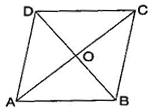Therefore, In {tex}\Delta {/tex}AOB, AB < OA + OB ……….(i)

In {tex}\Delta {/tex}BOC, BC < OB + OC ……….(ii)

In {tex}\Delta {/tex}COD, CD < OC + OD ……….(iii)

In {tex}\Delta {/tex}AOD, DA < OD + OA ……….(iv)

Adding eq. (i), (ii), (iii) and (iv),

AB + BC + CD + DA < OA + OB + OB + OC + OC + OD + OD + OA

{tex} \Rightarrow {/tex} AB + BC + CD + DA < 2OA + 2OB + 2OC + 2OD

{tex} \Rightarrow {/tex} AB + BC + CD + DA < 2[(AO + OC) + (DO + OB)]

{tex} \Rightarrow {/tex} AB + BC + CD + DA < 2(AC + BD)

Hence, it is proved.

NCERT Solutions for Class 7 Maths Exercise 6.4

###### Question 6.The lengths of two sides of a triangle are 12 cm and 15 cm. Between what two measures should the length of the third side fall?

Since, the sum of lengths of any two sides in a triangle should be greater than the length of third side.

It is given that two sides of triangle are 12 cm and 15 cm.

Therefore, the third side should be less than 12 + 15 = 27 cm.

And also the third side cannot be less than the difference of the two sides.

Therefore, the third side has to be more than 15 – 12 = 3 cm.

Therefore, the third side could be the length more than 3 cm and less than 27 cm.

## NCERT Solutions for Class 7 Maths Exercise 6.4

NCERT Solutions Class 7 Maths PDF (Download) Free from myCBSEguide app and myCBSEguide website. Ncert solution class 7 Maths includes text book solutions from Class 7 Maths Book . NCERT Solutions for CBSE Class 7 Maths have total 15 chapters. 7 Maths NCERT Solutions in PDF for free Download on our website. Ncert Maths class 7 solutions PDF and Maths ncert class 7 PDF solutions with latest modifications and as per the latest CBSE syllabus are only available in myCBSEguide.

## CBSE app for Students

To download NCERT Solutions for Class 7 Maths, Social Science Computer Science, Home Science, Hindi English, Maths Science do check myCBSEguide app or website. myCBSEguide provides sample papers with solution, test papers for chapter-wise practice, NCERT solutions, NCERT Exemplar solutions, quick revision notes for ready reference, CBSE guess papers and CBSE important question papers. Sample Paper all are made available through the best app for CBSE students and myCBSEguide website.Test Generator

Create question paper PDF and online tests with your own name & logo in minutes.myCBSEguide

Question Bank, Mock Tests, Exam Papers, NCERT Solutions, Sample Papers, Notes